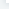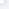# NumPy 排序、条件刷选函数

NumPy 排序、条件刷选函数NumPy 提供了多种排序的方法。 这些排序函数实现不同的排序算法，每个排序算法的特征在于执行速度，最坏情况性能，所需的工作空间和算法的稳定性。 下表显示了三种排序算法的比较。种类速度最坏情况工作空间稳定性'quicksort'（快速排序）1O(n^2)0否'mergesort'（归并排序）2O(n*log(n))~n/2是'heapsort'（堆排序）3O(n*log(n))0否numpy.sort()numpy.sort() 函数返回输入数组的排序副本。函数格式如下：numpy.sort(a, axis, kind, order)参数说明：a:

# 在 sort 函数中排序字段 dt = np.dtype([('name', 'S10'),('age', int)])a = np.array([("raju",21),("anil",25),("ravi", 17), ("amar",27)], dtype = dt)print ('我们的数组是：')print (a)print ('\n')print ('按 name 排序：')print (np.sort(a, order = 'name'))输出结果为：我们的数组是：[[3 7] [9 1]]调用 sort() 函数：[[3 7] [1 9]]按列排序：[[3 1] [9 7]]我们的数组是：[(b'raju', 21) (b'anil', 25) (b'ravi', 17) (b'amar', 27)]按 name 排序：[(b'amar', 27) (b'anil', 25) (b'raju', 21) (b'ravi', 17)]numpy.argsort()numpy.argsort() 函数返回的是数组值从小到大的索引值。实例import numpy as npx = np.array([3, 1, 2])print ('我们的数组是：')print (x)print ('\n')print ('对 x 调用 argsort() 函数：')y = np.argsort(x)print (y)print ('\n')print ('以排序后的顺序重构原数组：')print (x[y])print ('\n')print ('使用循环重构原数组：')for i in y:

print (x[i], end=" ")输出结果为：我们的数组是：[3 1 2]对 x 调用 argsort() 函数：[1 2 0]以排序后的顺序重构原数组：[1 2 3]使用循环重构原数组1 2 3numpy.lexsort()numpy.lexsort() 用于对多个序列进行排序。把它想象成对电子表格进行排序，每一列代表一个序列，排序时优先照顾靠后的列。这里举一个应用场景：小升初考试，重点班录取学生按照总成绩录取。在总成绩相同时，数学成绩高的优先录取，在总成绩和数学成绩都相同时，按照英语成绩录取…… 这里，总成绩排在电子表格的最后一列，数学成绩在倒数第二列，英语成绩在倒数第三列。实例import numpy as npnm = ('raju','anil','ravi','amar')dv = ('f.y.', 's.y.', 's.y.', 'f.y.')ind = np.lexsort((dv,nm))print ('调用 lexsort() 函数：')print (ind)print ('\n')print ('使用这个索引来获取排序后的数据：')print ([nm[i] + ", " + dv[i] for i in ind])输出结果为：调用 lexsort() 函数：[3 1 0 2]使用这个索引来获取排序后的数据：['amar, f.y.', 'anil, s.y.', 'raju, f.y.', 'ravi, s.y.']上面传入 np.lexsort 的是一个tuple，排序时首先排 nm，顺序为：amar、anil、raju、ravi 。综上排序结果为 [3 1 0 2]。msort、sort_complex、partition、argpartition函数描述msort(a)数组按第一个轴排序，返回排序后的数组副本。np.msort(a) 相等于 np.sort(a, axis=0)。sort_complex(a) 对复数按照先实部后虚部的顺序进行排序。partition(a, kth[, axis, kind, order]) 指定一个数，对数组进行分区argpartition(a, kth[, axis, kind, order]) 可以通过关键字 kind 指定算法沿着指定轴对数组进行分区复数排序：>>> import numpy as np>>> np.sort_complex([5, 3, 6, 2, 1])array([ 1.+0.j, 2.+0.j, 3.+0.j, 5.+0.j, 6.+0.j])>>>>>> np.sort_complex([1 + 2j, 2 - 1j, 3 - 2j, 3 - 3j, 3 + 5j])array([ 1.+2.j, 2.-1.j, 3.-3.j, 3.-2.j, 3.+5.j])partition() 分区排序：>>> a = np.array([3, 4, 2, 1])>>> np.partition(a, 3)

# 将数组 a 中所有元素（包括重复元素）从小到大排列，比第3小的放在前面，大的放在后面array([2, 1, 3, 4])>>>>>> np.partition(a, (1, 3))

# 小于 1 的在前面，大于 3 的在后面，1和3之间的在中间array([1, 2, 3, 4])找到数组的第 3 小（index=2）的值和第 2 大（index=-2）的值>>> arr = np.array([46, 57, 23, 39, 1, 10, 0, 120])>>> arr[np.argpartition(arr, 2)]10>>> arr[np.argpartition(arr, -2)[-2]]57同时找到第 3 和第 4 小的值。注意这里，用 [2,3] 同时将第 3 和第 4 小的排序好，然后可以分别通过下标  和  取得。>>> arr[np.argpartition(arr, [2,3])]10>>> arr[np.argpartition(arr, [2,3])]23numpy.argmax() 和 numpy.argmin()numpy.argmax() 和 numpy.argmin()函数分别沿给定轴返回最大和最小元素的索引。实例import numpy as npa = np.array([[30,40,70],[80,20,10],[50,90,60]])print ('我们的数组是：')print (a)print ('\n')print ('调用 argmax() 函数：')print (np.argmax(a))print ('\n')print ('展开数组：')print (a.flatten())print ('\n')print ('沿轴 0 的最大值索引：')maxindex = np.argmax(a, axis = 0)print (maxindex)print ('\n')print ('沿轴 1 的最大值索引：')maxindex = np.argmax(a, axis = 1)print (maxindex)print ('\n')print ('调用 argmin() 函数：')minindex = np.argmin(a)print (minindex)print ('\n')print ('展开数组中的最小值：')print (a.flatten()[minindex])print ('\n')print ('沿轴 0 的最小值索引：')minindex = np.argmin(a, axis = 0)print (minindex)print ('\n')print ('沿轴 1 的最小值索引：')minindex = np.argmin(a, axis = 1)print (minindex)输出结果为：我们的数组是：[[30 40 70] [80 20 10] [50 90 60]]调用 argmax() 函数：7展开数组：[30 40 70 80 20 10 50 90 60]沿轴 0 的最大值索引：[1 2 0]沿轴 1 的最大值索引：[2 0 1]调用 argmin() 函数：5展开数组中的最小值：10沿轴 0 的最小值索引：[0 1 1]沿轴 1 的最小值索引：[0 2 0]numpy.nonzero()numpy.nonzer

   下一页

NumPy 排序、条件刷选函数

## 相关文章